Data Structures
Lecture 01: Introduction to Algorithm Analysis
Edirlei Soares de LimaWhat is an Algorithm?
A set of instructions to solve a problem.
The problem is the motivation for the algorithm.
In most cases, there are several algorithms to solve the same problem.
How can we choose the algorithm?
Algorithms can be expressed using a variety of notations,
including natural language, pseudocode, flowcharts, or directly in
a programming language.
What algorithms are important for game programming?What is an Algorithm?
A computational problem is usually defined by a description
of its input and output.
Example: sorting
Input: a sequence {a1; a2; ... ; an} of n numbers
Output: a permutation of numbers {a'1; a'2; ... ; a’n} such
that a'1 a'2 ... a'n
Input: 6 3 7 9 2 4
Output: 2 3 4 6 7 9Effectiveness and Efficiency
Effectiveness: the algorithm must be able to
correctly solve all instances of the problem.
Efficiency: the performance of the algorithm (time
and space) must be appropriate to target application.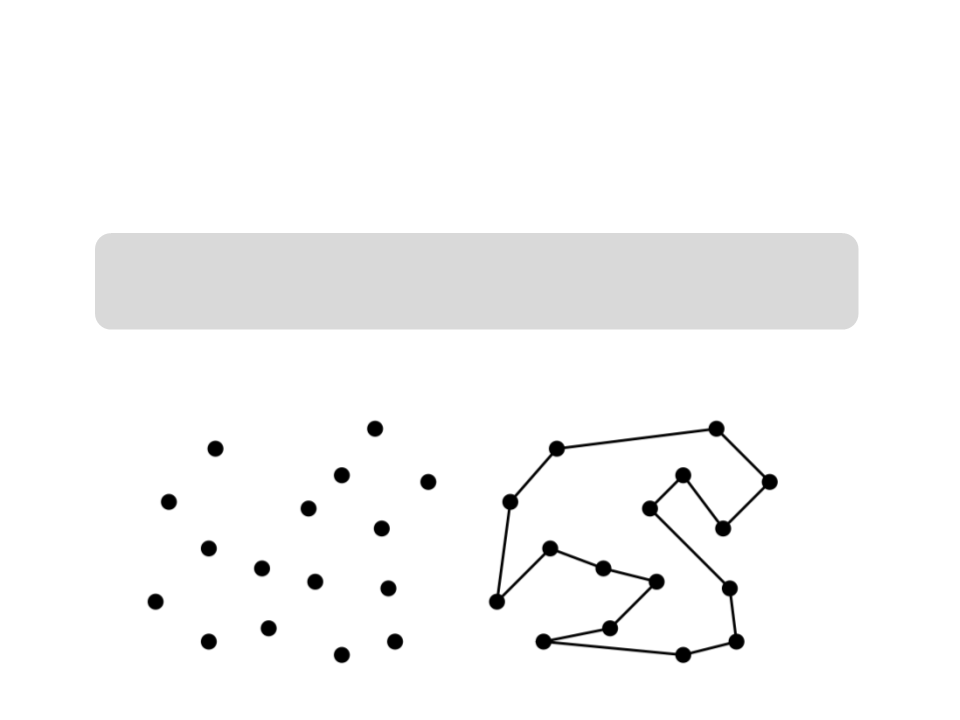Example: Effectiveness of an Algorithm
Traveling Salesman problem:
Input: a set S of n points in a plane
Output: the shortest cyclic path to visit all point of S
Example:Example: Effectiveness of an Algorithm
Traveling Salesman problem
The first possible algorithm:
1. p1 start point randomly selected
2
3
4
5
6
. i ← 1
. while (there are points to visit) do
. pi the closest not visited neighbor of pi
. ii + 1
. return path p1 p2 ...pn p1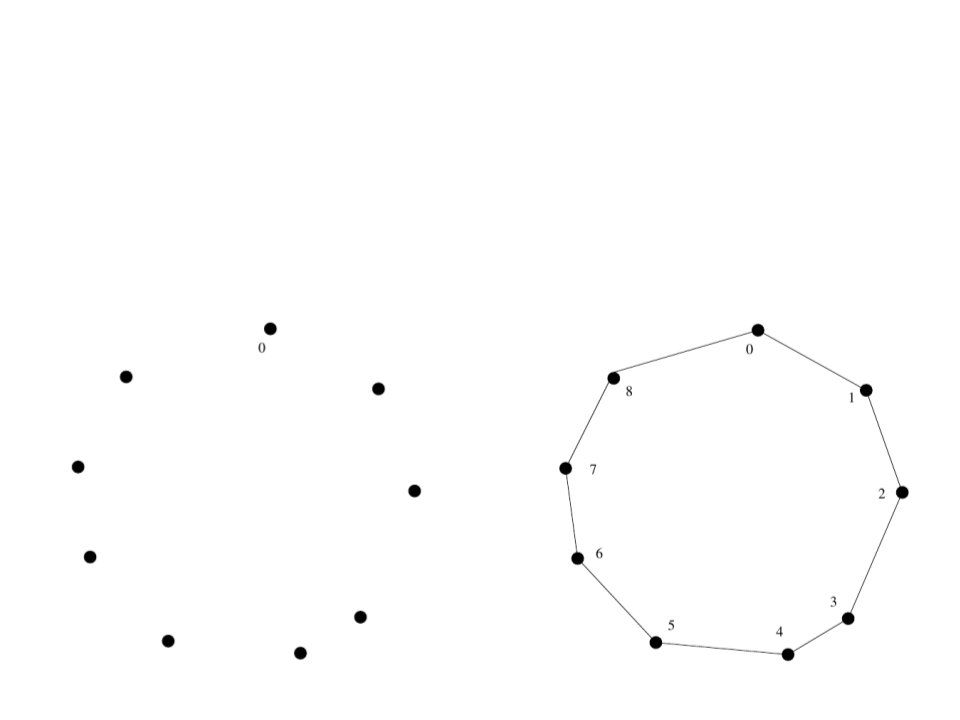Example: Effectiveness of an Algorithm
Traveling Salesman problem
It seems to work…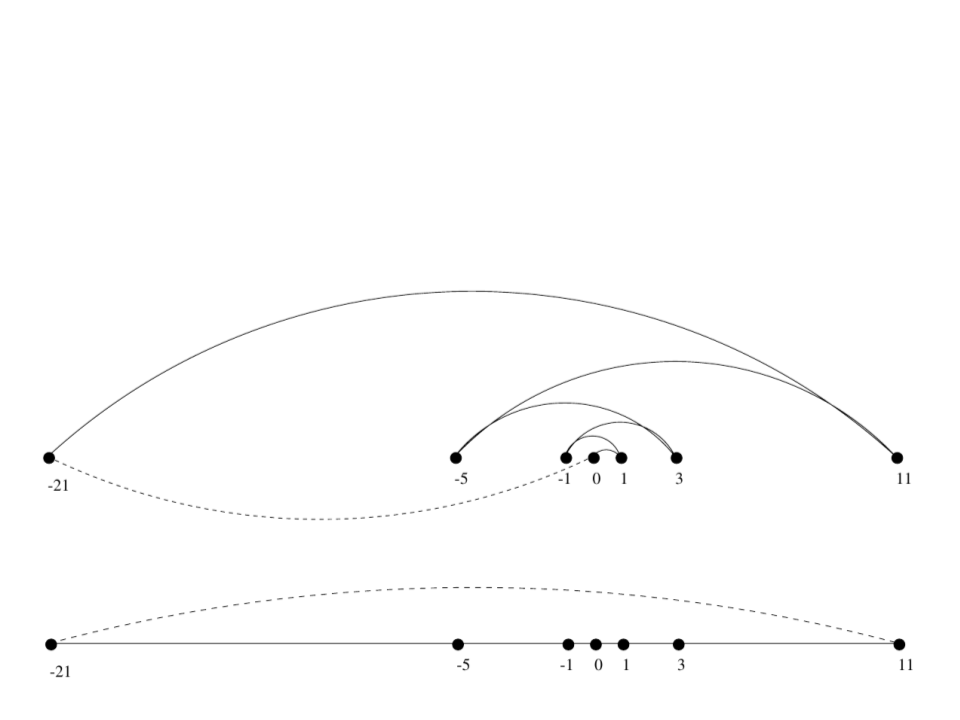Example: Effectiveness of an Algorithm
Traveling Salesman problem
But it does not work for all the instances of the problem!Example: Effectiveness of an Algorithm
Traveling Salesman problem
A second possible algorithm:
1. For i ← 1 to (n - 1) do
2
3
4
. Connect the closest pair of points that are part of
different connected components
. Connect the two points that are in the extremes of
the remaining connected component
. Return the cycle created with the pointsExample: Effectiveness of an Algorithm
Traveling Salesman problem
It seems to work…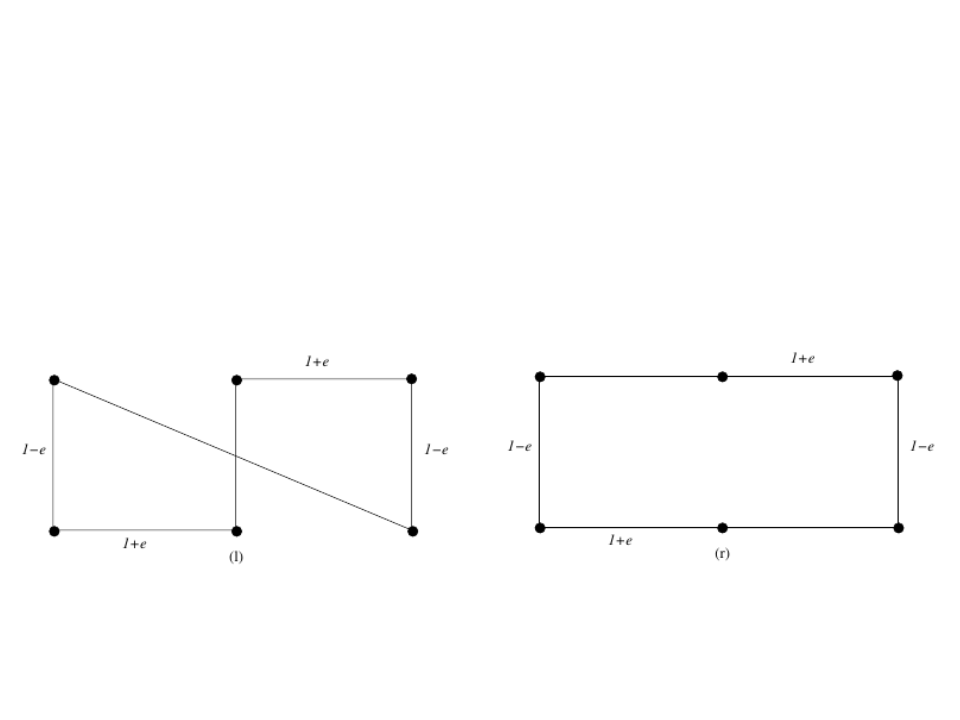Example: Effectiveness of an Algorithm
Traveling Salesman problem
But it does not work for all the instances of the problem!Example: Effectiveness of an Algorithm
Traveling Salesman problem
A third possible algorithm (brute force):
1. Pmin any permutation of S
2
3
4
5
. For Pi every permutation of S do
.
.
if (cost(Pi) < cost(Pmin)) then
Pmin Pi
. Return the path of Pmin
The algorithm is effective (correct), but extremely slow!
P(n) = n! = n × (n - 1) × ... × 1 (n factorial!)
Example: P(20) = 2,432,902,008,176,640,000Example: Effectiveness of an Algorithm
The Travelling Salesman Problem (TSP) is a classic problem in computer
science.
The problem has several applications that depend on its solutions.
Examples: industrial production, vehicle routes, genomic analysis ...
No efficient solution for this problem is known (some solutions generate
“approximated” results, but no one generates optimal results)
The temporal complexity of the brute force solution is: O(n!)
The TSP is part of class of problems known as NP-hard
There is decision version of the problem that is part of the class NP-complete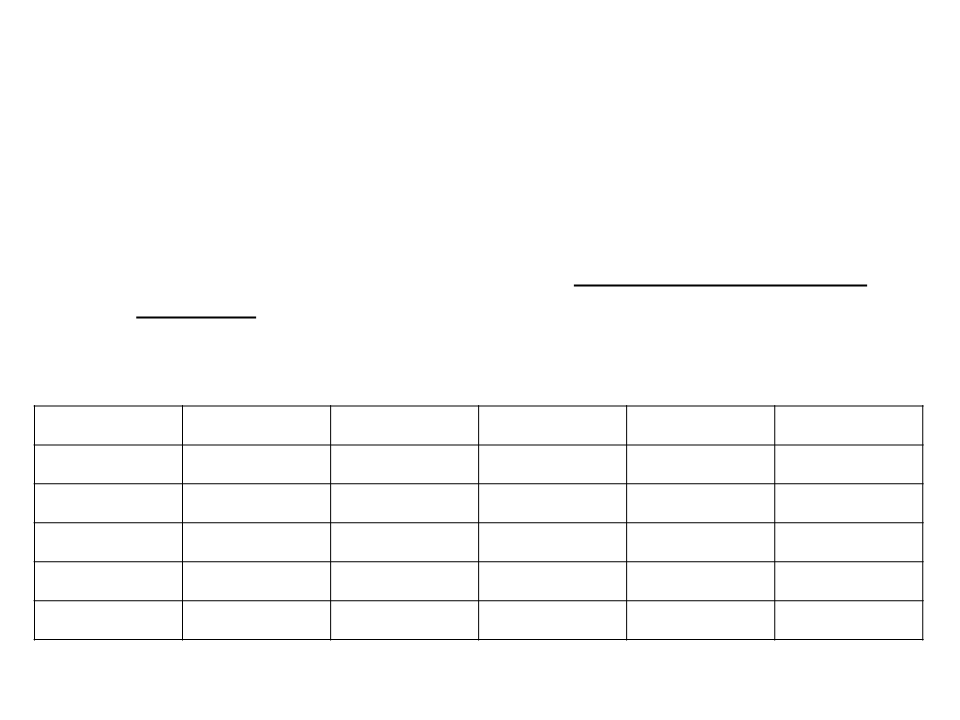Efficiency of an Algorithm
How many simple operations a computer can perform per
second? (just an approximation)
Supposing that a computer can perform 1 million simple operations
per second, how much time it would take to perform the following
sets of operations?
Operations
100
< 1 sec
< 1 sec
1 sec
1,000
< 1 sec
1 sec
10,000
< 1 sec
2 min
100,000
< 1 sec
1,000,000
1 sec
N
N2
N3
2N
N!
3 hours
32 years
12 days
18 min
12 days
31,710 years
1017 years
a lot of time a lot of time a lot of time a lot of time
a lot of time a lot of time a lot of time a lot of time a lot of time
a lot of time > 1025 yearsEfficiency of an Algorithm
Complexity of the Traveling Salesman brute force algorithm:
P(n) = n! = n × (n - 1) × ... × 1
Example of 6 permutations of {1, 2, 3}:
2 3
3 2
1
1
2
2
3
3
1 3
3 1
1 2
2 1Efficiency of an Algorithm
How much time the algorithm would need to check all the
permutations of n numbers? (approximated time considering
around 10 permutations per second)
7
n = 8: 0,003s
n = 9: 0,026s
n = 10: 0,236s
n = 11: 2,655s
n = 12: 33,923s
n = 20: 5000 years !Efficiency of an Algorithm
A faster computer can help?
if n = 20 = 5000 years, let’s use a faster computer:
10x faster would still need 500 years;
5,000x faster would need 1 year;
1,000,000x fast can solve the problem in 2 days… but:
n = 21 would take 1 month;
n = 22 would take 2 years!
The complexity growth of an algorithm is way more important
than a faster hardware!Efficiency of an Algorithm
How can we predict how much time an algorithm will
take to solve a problem?
How can we compare two different algorithms?Random Access Machine (RAM)
We need a model that is generic and independent of the
machine/language.
Random Access Machine (RAM)
Every simple operation (+, -, / , *) takes one time step;
All memory access takes exactly one time step;
Execution time: number of steps performed in a given input size (T(n)).
Simplified model (adding to integer numbers does not take
the same amount of time as dividing two float numbers, but
we will see that these differences do not affect the overall
estimation)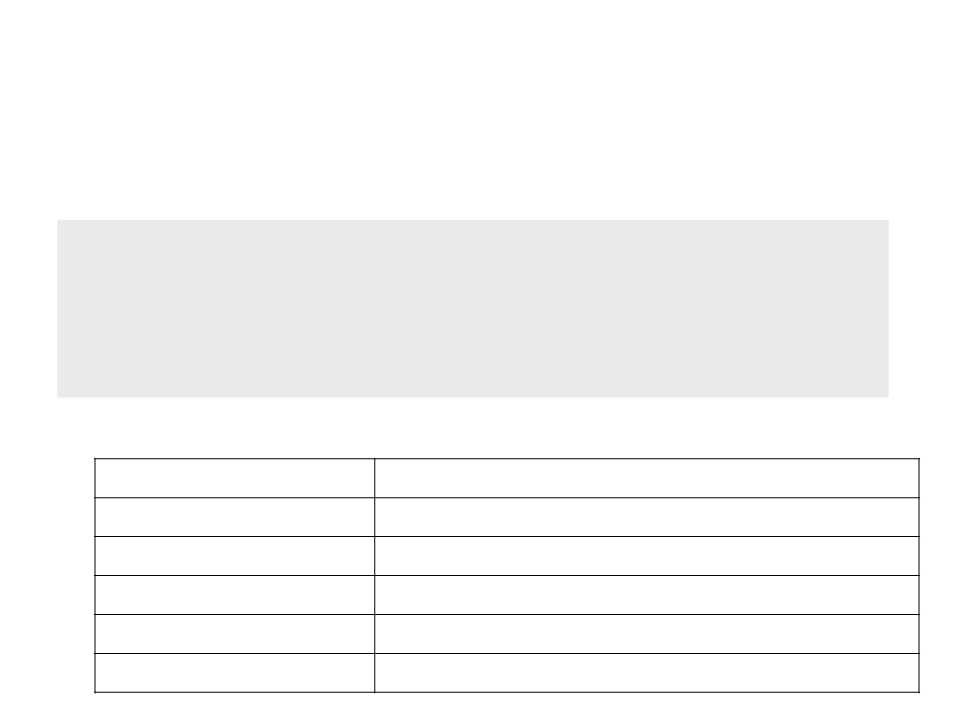Example: Number of Operations
A simple program:
int count = 0;
int i;
for (i=0; i<n; i++)
if (v[i] == 0)
count++;
Number of basic operations:
Variable declarations
Sums
2
2
Comparisons “less than”
Comparisons “equal”
Array accesses
n + 1
n
n
Increments
Between n and 2n (depending o the number of zeros)Example: Number of Operations
A simple program:
int count = 0;
int i;
for (i=0; i<n; i++)
if (v[i] == 0)
count++;
Total operations in the worst case:
T(n) = 2 + 2 + (n + 1) + n + n + 2n = 5 + 5n
Total operations in the best case:
T(n) = 2 + 2 + (n + 1) + n + n + n = 5 + 4nTypes of Algorithm Analysis
Worst Case Analysis:
T(n) = maximum time that an algorithm takes for any input
of size n.
Average Case Analysis:
T(n) = average time that an algorithm takes for all inputs of
size n.
Knowledge about the statistic distribution of the inputs is
necessary.
Best Case Analysis:
T(n) = naïve evaluation of the easiest inputs to be solved
by an algorithm.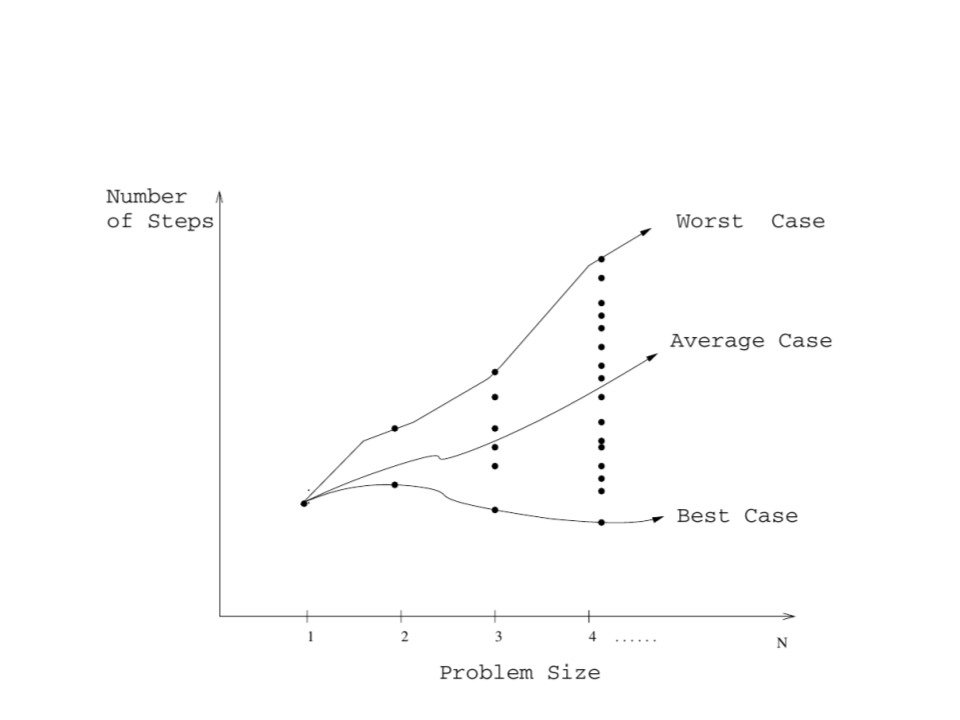Types of Algorithm AnalysisAsymptotic Analysis
To analyze algorithms, we need a mathematical method to
compare functions.
For algorithm analyzes, we use the Asymptotic Analysis
Study of the behavior of algorithms for arbitrarily large inputs
(complexity growth rate).
A specific notation is used: O, , (big O, omega, theta)
Allows us to "simplify" expressions focusing only on the
magnitude order.Asymptotic Analysis Notation
f(n) = O(g(n))
Means that C × g(n) describes the upper bound for f(n)
f(n) = (g(n))
Means that C × g(n) describes the lower bound for f(n)
f(n) = (g(n))
Means that C1 × g(n) describes the upper bound for f(n)
and C2 × g(n) describes the lower bound for f(n)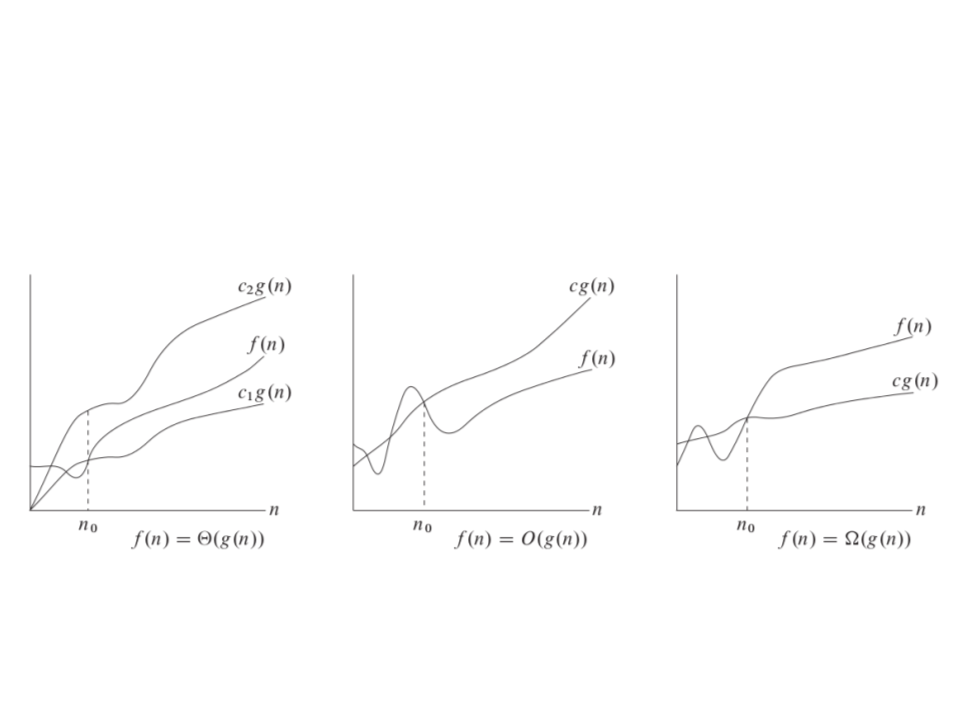Asymptotic Analysis Notation
OAsymptotic Analysis Formalization
f(n) = O(g(n)) if there are positive constants n0 and c
such that f(n) c × g(n) for every n n0
f(n) = 3n2 - 100n + 6
2
f(n) = O(n )
f(n) = O(n3)
f(n) O(n)Asymptotic Analysis Formalization
f(n) =(g(n)) if there are positive constants n0 and c
such that f(n) c × g(n) for every n n0
f(n) = 3n2 - 100n + 6
2
f(n) = Ω(n )
f(n) ≠ Ω(n3)
f(n) = Ω(n)Asymptotic Analysis Formalization
f(n) = (g(n)) if there are positive constants n0, c1 and
c2 such that c1 × g(n)f(n) c2 × g(n) for every n ≥
n0
f(n) = 3n2 - 100n + 6
2
f(n) = Θ(n )
f(n) ≠ Θ(n3)
f(n) ≠ Θ(n)
f(n) = Θ(g(n)) means that f(n) = O(g(n)) and f (n) = Ω(g(n))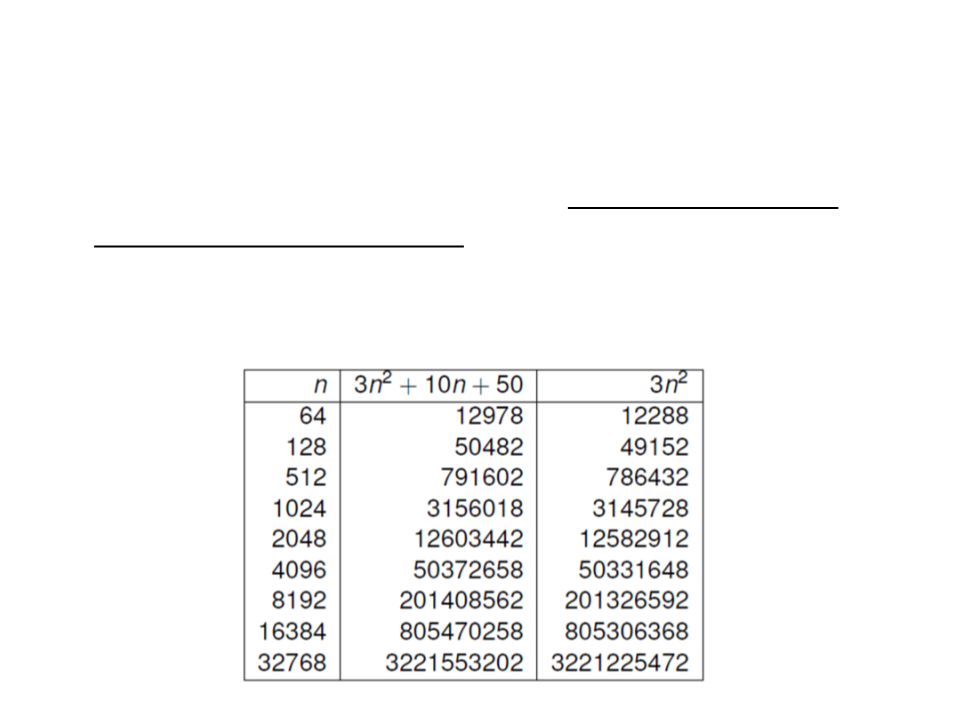Asymptotic Analysis
The asymptotic analysis allows us to ignore constants and
terms that are not dominant.
For example, considering the function: f(n) = 3n2 + 10n + 50Asymptotic Analysis Practical Rules
Multiplication by a constant does not change behavior of the
function:
Θ(c × f (n)) = Θ(f(n))
2 2
99 × n = Θ(n )
x
x-1
2
2
In a polynomial a n + a n + ... + a n + a1n + a0, we can focus on
x
x-1
the element with the highest exponent:
3 2 3
3n - 5n + 100 = Θ(n )
4 2 4
6n - 20 = Θ(n )
0.8n + 224 = Θ(n)
In an addition/subtraction, we can focus on the dominant element:
n
3
n
2 + 6n = Θ(2 )
2
n! - 3n = Θ(n!)
n log n + 3n = Θ(n2)
2Asymptotic Analysis Quick Exercise
T(n) = 32n2 + 17n + 32
T(n) is:
2
O(n )? Yes
3
O(n )? Yes
Ω(n)? Yes
2
Ω(n )? Yes
O(n)? No
2
Θ(n )? Yes
3
Ω(n )? No
Θ(n)? No
4
Θ(n )? No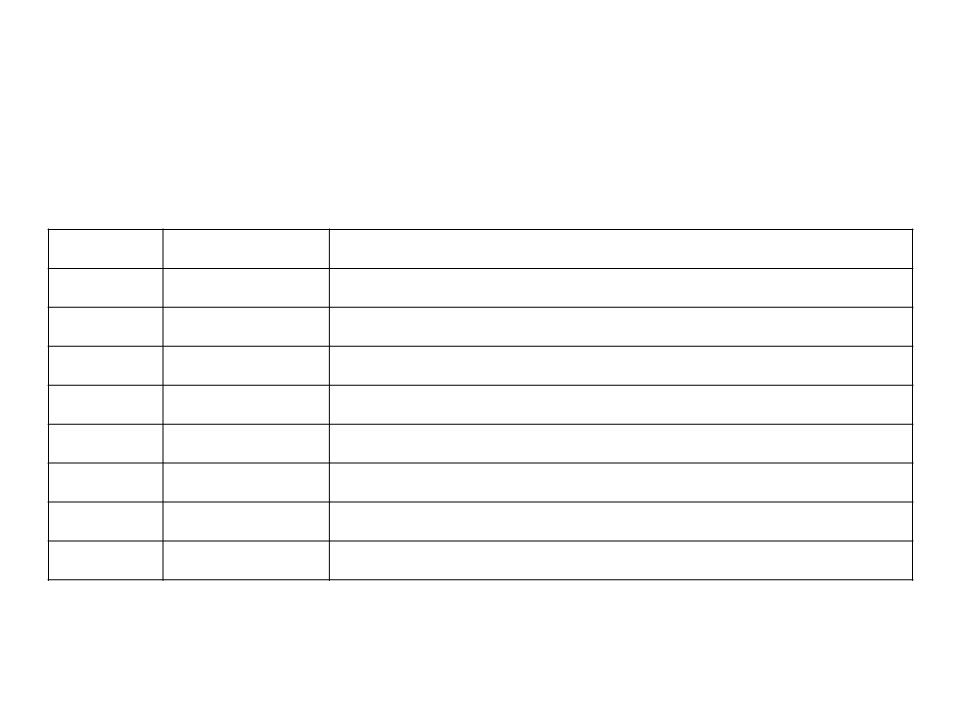Asymptotic Complexity Growth
Function Name
Examples
1
log n
n
constant
logarithmic
linear
Binary search, insert a value in a heap structure
Search a value in an array (one loop)
Sorting (merge sort, quick sort)
Sorting (bubble sort, selection sort) (two loops)
Floyd-Warshall (three loops)
n log n linearithymic
n2
n3
2n
n!
cubic
exponential
factorial
Exhaustive search in subsets
Generate all permutations of a set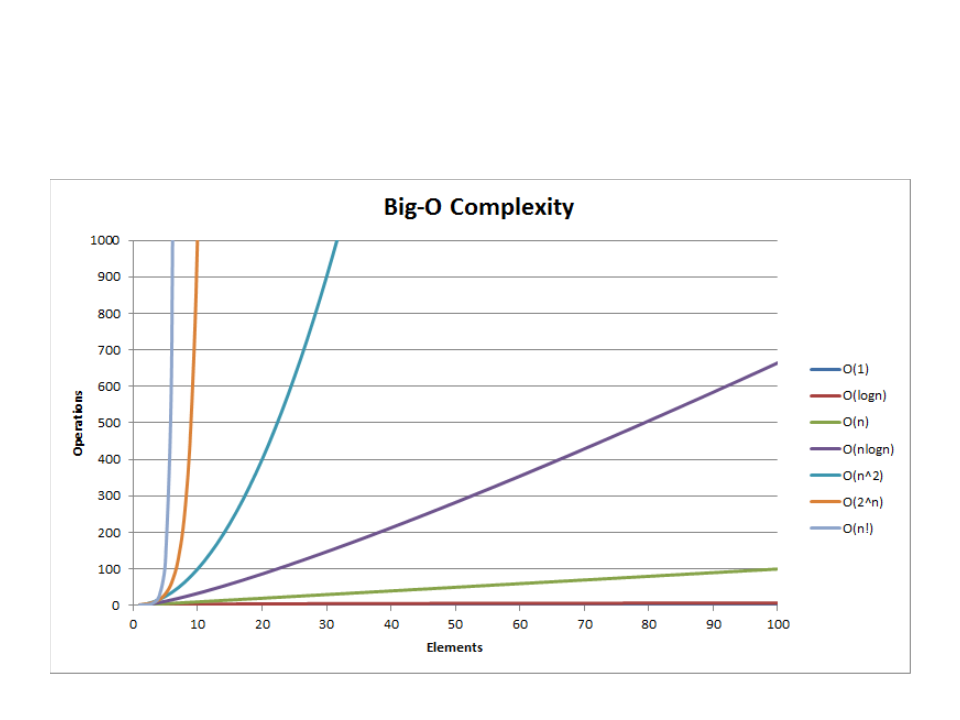Asymptotic Complexity GrowthAsymptotic Analysis Practical Examples
A program has two pieces of code A and B, executed one after the other,
2
with A running in O(n log n) and B in O(n ). What is the complexity of the
program?
2
O(n ) because n2 > n log n
A program calls an O(log n) function n times, and then calls another O(log
n) function again n times. What is the complexity of the program?
O(n log n)
A program has 5 loops, called sequentially, each with complexity O(n).
What is the complexity of the program?
O(n)
A program P has a running time proportional to 100 × n log n. Another
1
2
program P is 2 × n . What is the most efficient program?
1
2
2
P is more efficient because n > n log n.
However, for a small n, P is faster and it might make sense to have a program
2
that calls P or P according to the value of n.
1
2Quick Exercise 1
1
) Write an algorithm to check whether an array contains at
least two duplicate values (anywhere in the array). Then
analyze the complexity of the proposed algorithm.
bool hasDuplicate(int[] a){
int i, j;
for (i = 0; i < a.Length; i++){
for (j = 0; j < a.Length; j++){
if (i != j && a[i] == a[j]){
return true;
}
}
}
return false;
O(n2)
}Quick Exercise 2
) What is the complexity of the following algorithm?
2
int i, j, k, x, result = 0;
for (i = 0; i < N; i++){
for (j = i; j < N; j++){
for (k = 0; k < M; k++){
x = 0;
while (x < N){
m
result++;
x += 3;
n
n2
}
}
for (k = 0; k < 2 * M; k++)
if (k % 7 == 4)
m
result++;
}
O(n2mn) = O(mn3)
}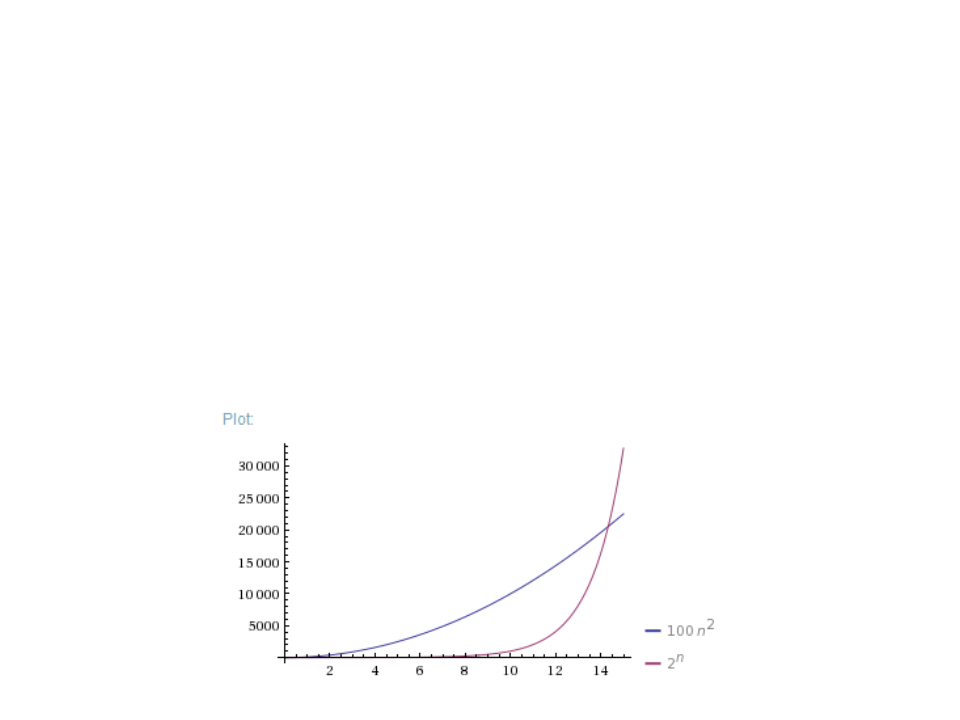Quick Exercise 3
3
) What is the smallest value of n such that an algorithm whose
2
execution time is 100n works faster than an algorithm whose
n
execution time is 2 on the same machine?
Wolfram Alpha:
plot 100*n^2, 2^n from n=0 to 15Assignment Algorithms
Assignment List 01 Algorithm Analysis
https://edirlei.com/aulas/data-structures/DS_Assignment_List_01.pdf